# Gray-Scale Operations

The easiest way, of course, to alter a digital image is to apply changes to its (usually 8-bit, from 0 to 255) gray-level values. Before we do this, it might be interesting to learn how the gray-level values of an image are distributed.

Histogram and Histograph

IMAQ Vision provides two simple tools for this distribution; they do almost the same thing:

• The histogram gives the numeric (quantitative) information about the distribution of the number of pixels per gray-level value.
• The histograph displays the histogram information in a waveform graph.

According to , the histogram function H (k) is simply defined as

Equation 4.1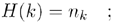with n k as the number of pixels with the gray-level value k . Figure 4.1 shows the histogram function H ( k ) for our bear image using IMAQ Vision Builder. In addition, you can try the following exercise.

Figure 4.1. Histogram Function in IMAQ Vision Builder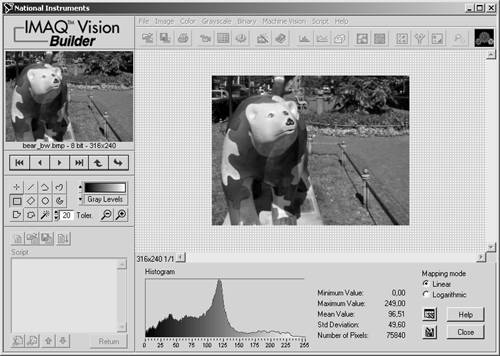Exercise 4.1: Histogram and Histograph.

Create a LabVIEW VI that provides the functionality described above directly in IMAQ Vision, using the functions IMAQ Histogram and IMAQ Histograph . You can find them under Motion and Vision / Image Processing / Analysis.  Figure 4.2 shows a possible solution.

 Actually, the histogram is an image analysis function according to our definition. On the other hand, we need its results for the following sections, so I explain it here.

From IMAQ Vision Builder, the histogram data can be exported for further processing into MS Excel; Figure 4.3 shows the data from Figure 4.1 and 4.2 in an MS Excel diagram.

Figure 4.2. Histogram and Histograph of an ImageFigure 4.3. Histogram Exported in MS ExcelIf the histogram function is applied to a color image, it returns values H ( k ) for each color plane; for example, three histograms for red, green, and blue in the case of an RGB image. Figure 4.4 shows an example using IMAQ Vision Builder.

Figure 4.4. Color Histogram Function in IMAQ Vision Builder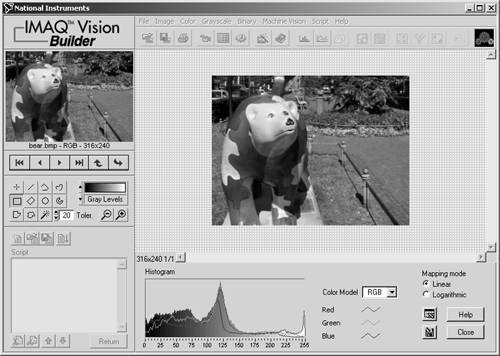Using Look-up Tables (LuT)

You know how to adjust brightness and contrast values of your TV set or TV video PC card; the intention is to get a "better" image, what obviously means a better distribution of the gray-level values. This is achieved by a function f ( g ) ( g are the gray-level values), which assigns a new value to each pixel.

If we consider Eq. (1.1), then our function f ( g ) will modify the gray-level values according to

Equation 4.2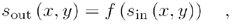with s in as the original values and s out as the resulting values. Because the possible results are limited to 256 values, the function f ( g ) usually is realized by a table consisting of 256 values, a look-up table (LuT). Therefore, if

Equation 4.3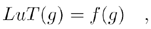Eq. (4.2) will change to

Equation 4.4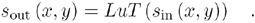Special LuTs: Math Functions

The easiest way to generate a LuT is to use mathematic functions, such as logarithmic , exponential, and power. We try this in the following exercise.

Exercise 4.2: Look-up Tables.

In this and the following exercise we compare the fixed IMAQ Vision functions to the impact of manually generated LuTs. First of all, create the VI shown in Figure 4.5.

Use the function IMAQ UserLookup to generate the second image, which is used for the comparison of the result to the built-in IMAQ function; here, the function IMAQ MathLookup . An XY graph displays the entire LuT by a simple line.

Figure 4.5. Exercise 4.2: Creating User LuTsFigure 4.6 shows the front panel of Exercise 4.2. Case 0 of the VI leaves the gray-level values of the original image unchanged, which means that the location 0 of the LuT gets the value 0, 1 gets 1, and so on until the location 255, which gets 255. The XY graph shows a line from bottom left to top right.

Figure 4.6. Processing Look-up Tables (LuTs)Another built-in LuT is a logarithmic one; if the function f ( g ) were f ( g ) = log( g ), then it would be sufficient to add the logarithm base 10 function of LabVIEW to case 1 and watch the results.

Oops ”that did not work. Naturally, the logarithm (base 10) of 255 is about 2.4, so we have to scale the entire range of resulting values to the 8-bit gray-scale set. The resulting function is

Equation 4.5and is shown in Figure 4.7.

Figure 4.7. Creating a Logarithmic Look-up Table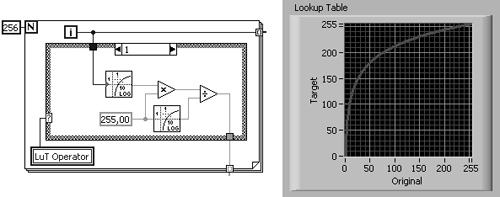The next function is exponential. If we generate it the way we did above, we see a far too steep function because the values rise too fast. A correction factor c , according to

Equation 4.6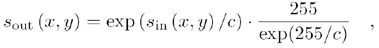leads to more reasonable results. c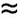48 is the value used in the built-in IMAQ function (see the realization of this equation in Figure 4.8).

Figure 4.8. Creating an Exponential Look-up Table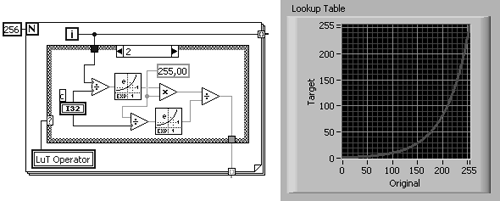The square function is easy: If we multiply the value location by itself and scale it like we did above, we get

Equation 4.7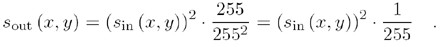Figure 4.9 shows the corresponding results.

Figure 4.9. Creating a Square Look-up TableSquare root is quite similar. Figure 4.10 shows the results, and here is the function:

Equation 4.8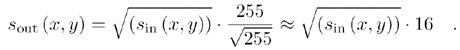Figure 4.10. Creating a Square Root Look-up TableThe next two cases need an additional factor called power value p : We create the function

Equation 4.9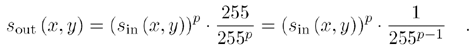IMAQ Vision calls this function power x ; its realization can be seen in Figure 4.11 for a power value of p = 3.

Figure 4.11. Creating a Power x Look-up TableThe last function used in this exercise is called power 1/ x and is simply realized by calculating the reciprocal value of p ; use it like the power x function:

Equation 4.10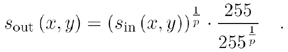Figure 4.12 shows the implementation of the power 1/ x function in LabVIEW and IMAQ Vision.

Figure 4.12. Creating a Power 1/ x Look-up Table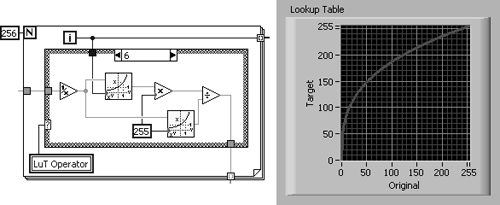It is easy to see that these functions can be divided into two groups: Functions like logarithmic, square root, and power 1/ x compress regions with high gray-level values and expand regions with low gray-level values. The exponential, square, and power x functions operate conversely; they expand regions with high gray-level values and compress regions with low gray-level values.

The power value p is often called gamma coefficient; it indicates the degree of impact of the power function. We return to it in Exercise 4.5.

Special LuTs: Equalize and Inverse

IMAQ Vision also contains a function called IMAQ Equalize , which distributes the gray-level values evenly over the entire gray-scale set (usually 0 to 255 for 8 bits). If an image does not use all available gray levels (most images do not), the contrast (the difference from one gray level to another) of the image can be increased.

Exercise 4.3: Equalizing Images.

Create a VI that equalizes our bear image. In this case, it is not so easy to create a user defined LuT, so we concentrate on the histograms. Both of them can be seen in Figure 4.13; Figure 4.14 shows the diagram.

The histogram of the equalized image in Figure 4.13 is quite interesting. It is obviously not a solid line like the histogram of the original image. This is because the equalize function distributes an equal amount of pixels over a constant gray-level interval. If not all gray-level values are used in the original image, the histogram of the resulting image contains values without pixels.

Figure 4.13. Image and Histogram Resulting from Equalizing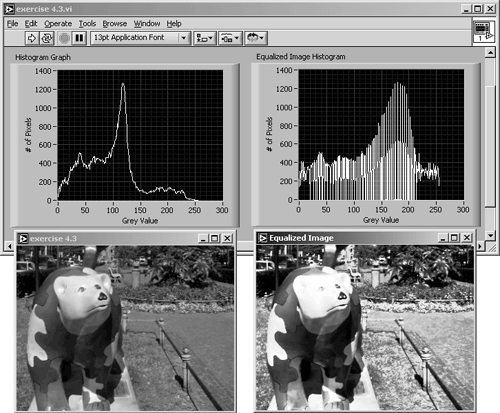Figure 4.14. Diagram of Exercise 4.3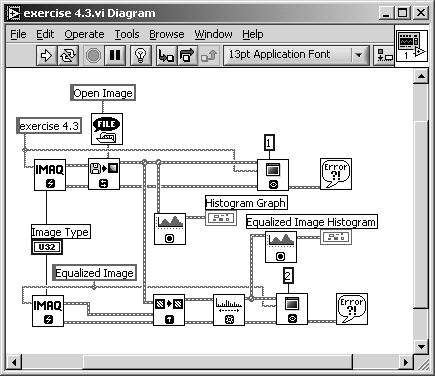The next function is very simple: inverse. High gray-level values are replaced by low gray-level values, and vice versa: 0 becomes 255, 1 becomes 254, and so forth until 255, which becomes 0. This procedure can be described by

Equation 4.11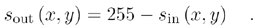Exercise 4.4: Manual Creation of LuTs.

In this exercise we create the LuT manually by simply subtracting the actual value from 255, as in Eq. (4.11). The resulting images and the LuT are shown in Figure 4.15; the diagram is shown in Figure 4.16.

Figure 4.15. Inverting the Bear Image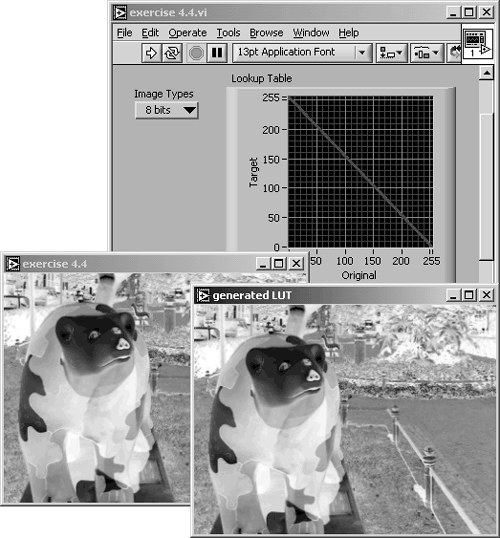Figure 4.16. Diagram of Exercise 4.4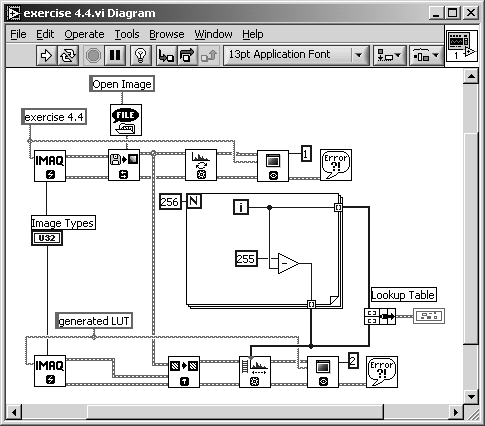Now you know why you should plot the LuTs in an XY graph. In most cases, you can predict the results by simply looking at the plotted LuT. For example, if the LuT graph rises from bottom left to top right, then the resulting image will be not inverted; if it looks like a logarithmic function, the overall brightness of the resulting image will be increased because the log function compresses regions of high gray-level values.

Special LuTs: BCG Values

Another method is shown in Exercise 4.5. Instead of creating the LuT by using mathematical functions, we can use the function IMAQ BCGLookup , which changes the following parameters:

• Brightness: the median value of the gray-level values. If the LuT is drawn as a line as in the previous exercises, changing the brightness value shifts the line to the top (lighter) or to the bottom ( darker ).
• Contrast: the difference from one gray-level value to another. Varying the contrast value changes the slope of the line in the XY graph.
• Gamma: the gamma coefficient is equal to the power factor p we used in the previous exercises. If the gamma value is equal to 1, the graph always remains a straight line.

Exercise 4.5 compares the IMAQ function with a self- constructed one:

Exercise 4.5: BCG Look-up Table.

The IMAQ function IMAQ BCGLookup modifies the image by changing the values of brightness, contrast, and gamma. Create a VI that does the same with a manually generated LuT.

Figure 4.17 shows a possible solution; Figure 4.18 shows the diagram. I did only the brightness and the contrast adjustment; the realization of the gamma coefficient is left for you.

Figure 4.17. Using Special LuTs for Modifying Brightness and ContrastFigure 4.18. Diagram of Exercise 4.5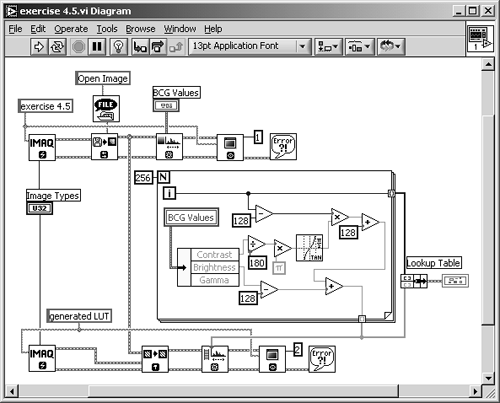### Spatial Image Filtering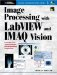Image Processing with LabVIEW and IMAQ Vision
ISBN: 0130474150
EAN: 2147483647
Year: 2005
Pages: 55